# Bayes Estimators for the Continuous Uniform Distribution

Allan J. Rossman
Dickinson College

Thomas H. Short
Villanova University

Matthew T. Parks
Boston University

Journal of Statistics Education v.6, n.3 (1998)

Copyright (c) 1998 by Allan J. Rossman, Thomas H. Short, and Matthew T. Parks, all rights reserved. This text may be freely shared among individuals, but it may not be republished in any medium without express written consent from the authors and advance notification of the editor.

Key Words: Highest posterior density interval; Improper prior distribution.

## Abstract

Classical estimators for the parameter of a uniform distribution on the interval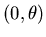are often discussed in mathematical statistics courses, but students are frequently left wondering how to distinguish which among the variety of classical estimators are better than the others. We show how classical estimators can be derived as Bayes estimators from a family of improper prior distributions. We believe that linking the estimation criteria in a Bayesian framework is of value to students in a mathematical statistics course, and we believe that the students benefit from the exposure to Bayesian methods. In addition, we compare classical and Bayesian interval estimators for the parameter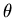and illustrate the Bayesian analysis with an example.

# 1. Introduction

1 The continuous uniform distribution is widely studied in mathematical statistics textbooks and courses in part because classical estimation criteria produce different estimators for the parameter. Lettinghave independent uniform distributions on the interval, the likelihood function is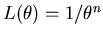for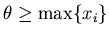.

2 The maximum likelihood estimator ofis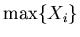, while the minimum variance unbiased estimator is. Furthermore, among estimators of the form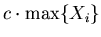, the one which minimizes the mean squared error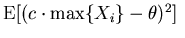is. These results can be found in many textbooks on mathematical statistics, including Freund (1992), Hogg and Craig (1978), and Larsen and Marx (1986).

3 While we find this example useful for helping students discover that classical estimation criteria can in fact lead to different estimators, we nevertheless feel a sense of unease when students naturally ask which estimator is better.'' At this point we are tempted to turn from the competing desirability criteria of the classical approach to the unifying philosophy and analysis strategy of a Bayesian framework. As we will show, this example is ideal in that a Bayesian analysis with a simple family of improper prior distributions provides a direct link among several classical estimators.

4 Moreover, we contend that students of mathematical statistics should explore principles of Bayesian inference for a variety of reasons. One is that the development and use of Bayesian methods are on the increase. A growing number of papers appearing in statistical forums such as the Journal of the American Statistical Association represent the Bayesian approach, and even some applied statisticians have adopted a Bayesian viewpoint. The American Statistician recently presented a collection of papers by Berry (1997), Moore (1997), and Albert (1997), with accompanying discussion, exploring the value of a Bayesian perspective in an introductory statistics course.

5 A second reason for encouraging students to study the Bayesian paradigm is that it models the process of science. Berry (1997) writes that science progresses with scientists altering their opinions as information accumulates, and with scientists trying to persuade other scientists of the correctness of their opinions.'' Eliciting opinions, updating after observing data, and quantifying uncertainty using probability distributions are all part of Bayesian statistics.

6 A third motivation for studying Bayesian statistics is that students might better understand classical procedures and estimation criteria by studying them in comparison to Bayesian methods.

# 2. Bayesian Analysis

7 Few undergraduate texts present a Bayesian analysis of the continuous uniform distribution, although DeGroot (1986), Lee (1989), and DeGroot (1970) present the Pareto distribution as a conjugate family of prior distributions. One can adopt a simpler form for the prior distribution by considering improper priors which do not integrate to one but still perform the same function as a proper prior distribution. For instance, if one chooses the flat improper prior distribution of the form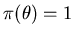for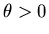, the posterior distribution is proportional to the likelihood function,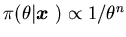for. This posterior distribution is proper provided that n > 1, with the constant of proportionality turning out to be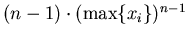. Assuming a quadratic loss function, the Bayes estimator equals the posterior mean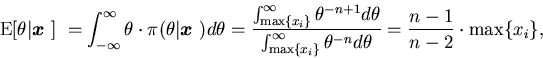which exists when n > 2. This Bayesian analysis produces yet another estimator which equals a constant times the sample maximum, where the constant has the form (n + m) / (n + m - 1) for some m and approaches 1 as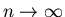.

8 In fact, one can derive all estimators of this form from a Bayesian perspective. Consider the family of prior distributions having the form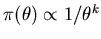for. These distributions are improper for any real k. The resulting posterior distribution is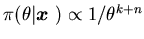for, which is proper when k + n > 1 with the constant of proportionality equaling. The posterior mean exists when k + n > 2, producing a Bayes estimator of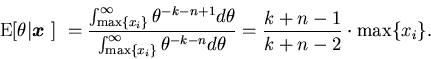Notice that this estimator corresponds to the minimum variance unbiased estimator when k = 2 and to the minimum mean square error estimator when k = 3. Choosing k = 1 yields the estimator, which seems to be missing in the sequence of estimators above. Thus, the estimators ofthat emerge from various classical criteria of estimation can be seen as members of a sequence of Bayes estimators based on this family of improper prior distributions.

9 Positive values of k can be interpreted to represent k unobserved uniform random variables on the interval. Larger values of k put more prior weight on smaller values ofand therefore produce lower posterior estimates.

10 One can also compare classical and Bayesian interval estimators of the parameter. The classical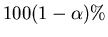confidence interval forissince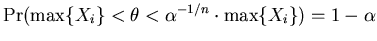. From the Bayesian perspective, ahighest probability density (HPD) interval for, using the family of improper prior distributions described above, turns out to be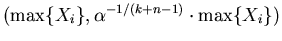since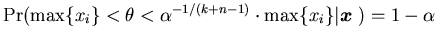. The classical and Bayesian interval estimators are therefore the same when k = 1.

11 The choice of k = 1 comes highly recommended from the Bayesian literature because it corresponds to the Jeffreys' prior, which is in this case a standard noninformative prior distribution for a scale parameter. The Jeffreys' prior is noninformative because it is invariant to parameter transformations. For example,may be transformed to obtain standard deviationor variance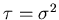. The prior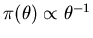is equivalent to priors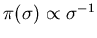oron the standard deviation or scale parameters, respectively. Furthermore,is noninformative on the ratio scale -- for a given constant c, it implies that all intervals of the form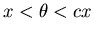are equally likely for any choice of x. See, for example, Box and Tiao (1973) for more information about Jeffreys' priors.

12 Larger values of k in the prior distribution represent increased prior certainty about the value of the parameter, and thus produce narrower posterior HPD intervals.

# 3. Example

13 As an example suppose that n = 12 and that the observed data are:

x = (2.6, 2.8, 3.6, 4.3, 5.5, 10.3, 12.2, 20.2, 21.8, 28.7, 30.6, 32.2).

Starting with a flat improper prior distribution forcorresponding to k = 0 produces the posterior distribution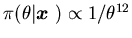for> 32.2, which is displayed in Figure 1. Note that the height of the improper prior distribution displayed in Figure 1 is arbitrary. The Bayes estimate ofis (11/10) � 32.2 = 35.42, and a 95% posterior HPD interval foris (32.2, (.05)-1/11 � 32.2) = (32.2, 42.28). For the sake of comparison, Table 1 lists Bayes estimates and interval estimates offor other values of k and points out their classical counterparts. Figure 2 graphs Bayes estimates and HPD interval upper bounds as continuous functions of k, and also indicates values that correspond to estimates based on classical criteria.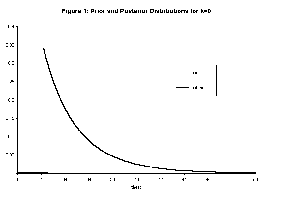Figure 1 (6.0K gif)

Figure 1. Prior and Posterior Distributions for k = 0.

Table 1. Bayes Estimates for Various Values of k

 k Bayes estimate(posterior mean) Upper bound of95% HPD interval Bayesianinterpretation Classicalinterpretation -2 36.23 44.92 -1 35.78 43.45 0 35.42 42.28 flat prior 1 35.13 41.33 Jeffreys' prior confidence interval 2 34.88 40.55 unbiased estimate 3 34.68 39.88 minimum MSE estimate 4 34.50 39.32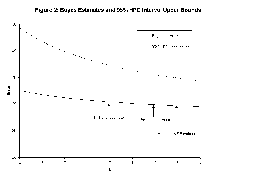Figure 2 (6.2K gif)

Figure 2. Bayes Estimates and 95% HPD Interval Upper Bounds.

# 4. Conclusion

14 We have demonstrated that a Bayesian framework unites the various classical estimators produced by different estimation criteria for the parameter of a continuous uniform distribution. The Bayes estimators arise from a family of improper prior distributions and highlight both differences and similarities of Bayesian and classical analyses.

15 We believe that this comparison can help students of mathematical statistics both to gain valuable experience with Bayesian methods and also to understand classical estimation criteria more fully.

## Acknowledgments

The authors thank Jerry Moreno, Jeff Witmer, three anonymous referees, and the editor for comments that improved the quality of this article.

# References

Albert, J. (1997), "Teaching Bayes' Rule: A Data-Oriented Approach," The American Statistician, 51, 247-253.

Berry, D. A. (1997), "Teaching Elementary Bayesian Statistics with Real Applications in Science," The American Statistician, 51, 241-246.

Box, G. E. P., and Tiao, G. C. (1973), Bayesian Inference in Statistical Analysis, New York: John Wiley and Sons, Inc.

DeGroot, M. H. (1970), Optimal Statistical Decisions, New York: McGraw-Hill, Inc.

----- (1986), Probability and Statistics (2nd ed.), Reading, MA: Addison-Wesley Publishing Company.

Freund, J. E. (1992), Mathematical Statistics (5th ed.), Englewood Cliffs, NJ: Prentice Hall.

Hogg, R. V., and Craig, A. T. (1978), Introduction to Mathematical Statistics (4th ed.), New York: Macmillan Publishing Co., Inc.

Larsen, R. J., and Marx, M. L. (1986), An Introduction to Mathematical Statistics and Its Applications (2nd ed.), Englewood Cliffs, NJ: Prentice Hall.

Lee, P. M. (1989), Bayesian Statistics: An Introduction, New York: Oxford University Press.

Moore, D. S. (1997), "Bayes for Beginners? Some Reasons to Hesitate," The American Statistician, 51, 247-253.

Allan J. Rossman
Department of Mathematics and Computer Science
Dickinson College
Carlisle, PA 17013

rossman@dickinson.edu

Thomas H. Short
Department of Mathematical Sciences
Villanova University
Villanova, PA 19085

short@monet.vill.edu

Matthew T. Parks
Department of Political Science
Boston University
Boston, MA 02215

mparks@bu.edu